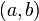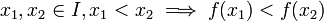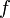# Increasing and differentiable implies nonnegative derivative that is not identically zero on any interval

## Statement

### On an open interval

Suppose$f$ is a function on an open interval$I$ that may be infinite in one or both directions (i..e,$I$ is of the form$\! (a,b)$,$(a,\infty)$,$(-\infty,b)$, or$(-\infty,\infty)$). Suppose the derivative of$f$ exists everywhere on$I$. Suppose further that$f$ is an increasing function on$I$, i.e.:$x_1,x_2 \in I, x_1 < x_2 \implies f(x_1) < f(x_2)$

Then,$\! f'(x) \ge 0$ for all$x \in I$. Further, there is no sub-interval of$I$ such that$\! f'(x) = 0$ for all$x$ in the sub-interval.

### On a general interval

Suppose$f$ is a function on an interval$I$ that may be infinite in one or both directions and may be open or closed at either end. Suppose$f$ is a continuous function on all of$I$ and that the derivative of$f$ exists everywhere on the interior of$I$. Further, suppose$f$ is an increasing function on$I$, i.e.:$x_1,x_2 \in I, x_1 < x_2 \implies f(x_1) < f(x_2)$

Then,$\! f'(x) \ge 0$ for all$x$ in the interior of$I$. Further, there is no sub-interval of$I$ such that$\! f'(x) = 0$ for all$x$ in the sub-interval.

## Proof

If$\! f$ is increasing on$I$, then every point in the interior of$I$ is a point of local maximum from the left and local minimum from the right. Thus, by Facts (1) and (2), both the left hand derivative and the right hand derivative of$f$, if they exist, are nonnegative at any point in the interior of$I$. In particular, if the derivative itself exists at a point in the interior of$I$, then it must be nonnegative at that point.

It remains to show that the derivative is not zero on any sub-interval of$I$. For this, note that by Fact (3), a derivative of zero forces the function to be constant on that sub-interval. This, however, contradicts the definition of an increasing function. We thus have the desired contradiction and we are done.